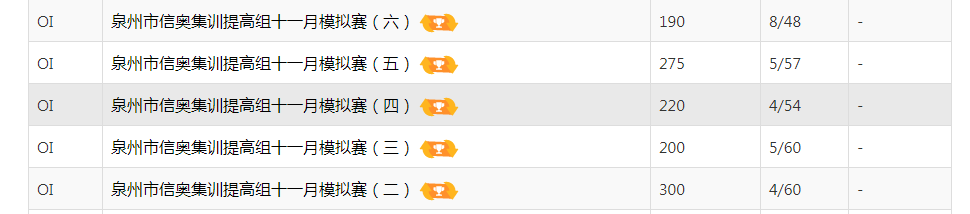# Day -1# Day 1

## 进考场

$T1$ 是个模拟，$T2$第一眼看不太出来，$T3$应该是数据结构题，$T4$应该是个性质或者结论题叭

$WA$了，它$WA$了。

$100pts$到手回去继续调$T1$,开始调调调调调调调调调调调调调调调调调调调调调调调调调调调调调调，调了大概$5$点半，没调出来，只好去写$T3$暴力了，打完暴力大概$5$点50，现在$145$到手了

$5min$打了$T4$暴力。

# Day 2

$luogu$上测了一下 $0 + 95 + 25 + 0 = 120$

$T1$挂到$0$分也总归是自己的问题吧

posted @ 2020-11-08 20:38  Dix-  阅读(140)  评论(0编辑  收藏